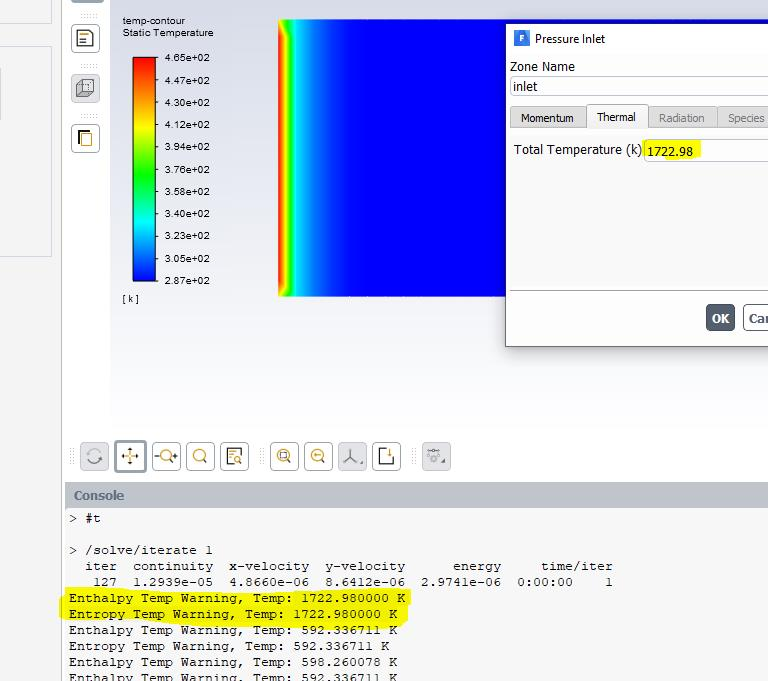CaptainConvergence
Subscriber

I am running a 2D channel where the static temperature is 287 at Mach 5. In order to have that static temperature, I need a total temperature of 1722.98 Kelvin at the inlet and outlet. My UDFs for Enthalpy and Entropy look like this:

/*

*/
/*
*/
/* ENTHALPY UDF */
/*
*/
/*
*/
double REAL_enthalpy(cell_t cell, Thread *thread, double Temp, double density, double P, double yi[])
{
double cp = REAL_specific_heat(cell, thread, Temp, density, P, yi); /* Cp = 1004.16 J/(Kg K) IF Temp < 500 Kelvin*/
double h = Temp * cp;
if (Temp>500){
Message("Enthalpy Temp Warning, Temp: %f Kn", Temp);
}

return h; /* (J/Kg) */
}
/*
*/
/*
*/
/* ENTROPY UDF */
/*
*/
/*
*/

double REAL_entropy(cell_t cell, Thread *thread, double Temp, double density, double P, double yi[])
{
double RGAS = Runiv/MW0;
double s = REAL_specific_heat(cell, thread, Temp, density, P, yi) * log(fabs(Temp / TDatum)) + RGAS * log(fabs(PDatum / P));
if (Temp>500){
Message("Entropy Temp Warning, Temp: %f Kn", Temp);
}

return s; /* (J/Kg/K) */
}

I have attached one screeshot 1 iteration after the initialization with a temperature of 287K. As you can observe, the messages that I set inform me that the variable Temp passed from the solver is 1722.98 Kelvin which is the total temperature and it is used to calculate the CP in the enthalpy function. Since the Cp function in the enthalpy function is given the Total temperature instead of the static temperature, the outcome is a highter Cp and thus a higher enthalpy that raises my static temperature from 287 Kelvin to 465 Kelvin.